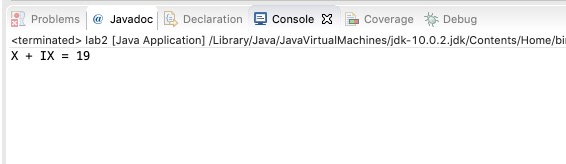# Lab 2

## Figure 1: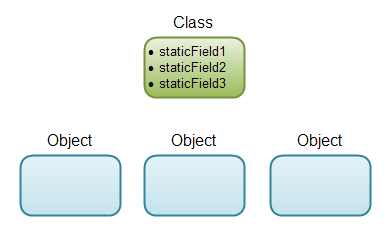In this lab we're going to learn more about the difference between static and object classes. Before we start lets create a new Java class for this lab. Follow the instructions below:

1. Open Eclipse and highlight the src folder under the Java project labs that we created in Lab 1
2.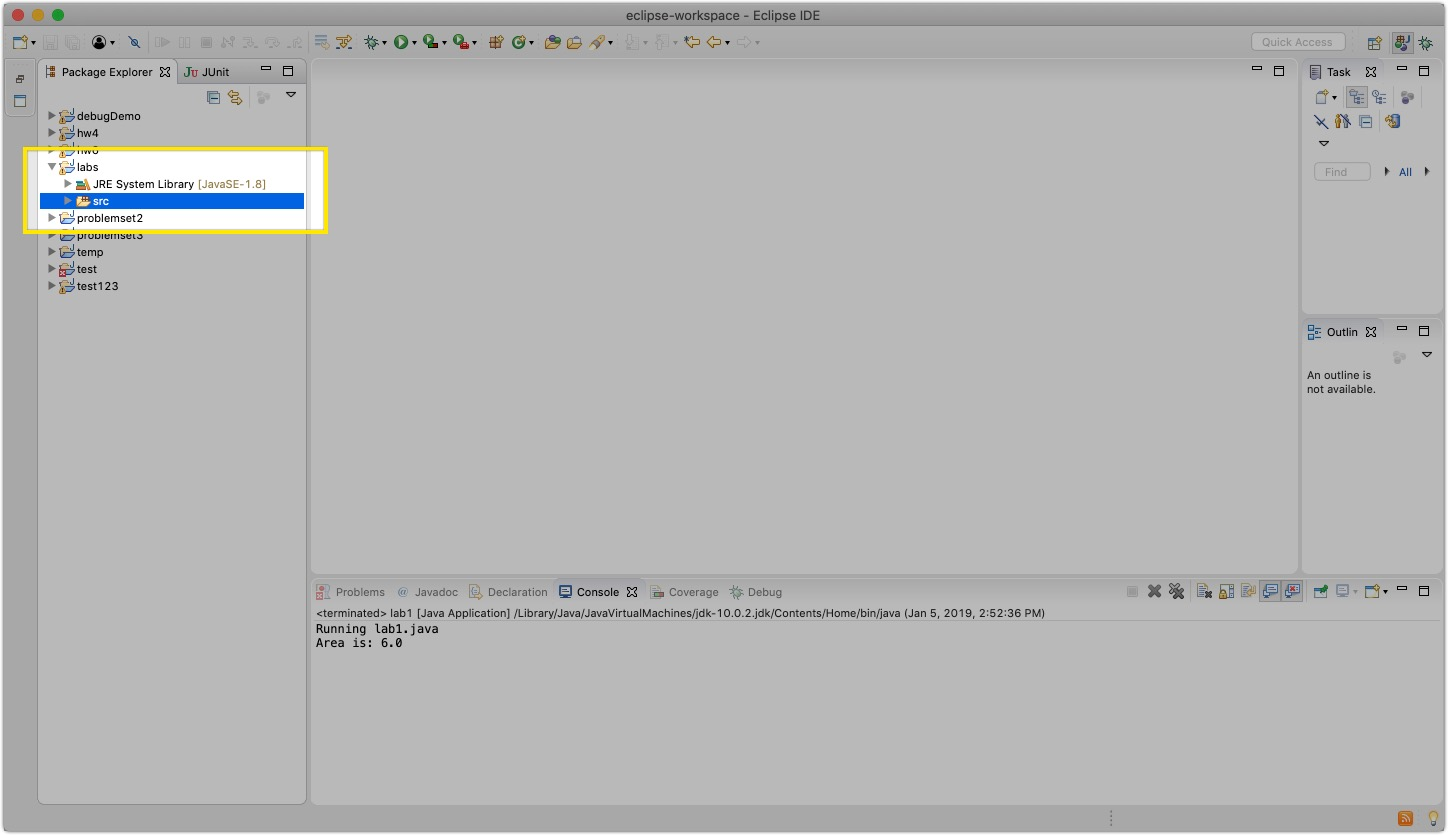3. Create a new class like we did in Lab 1
4.5. Exactly like we did in Lab 1, make sure to name the file lab2, select the appropriate information and click finish.
6.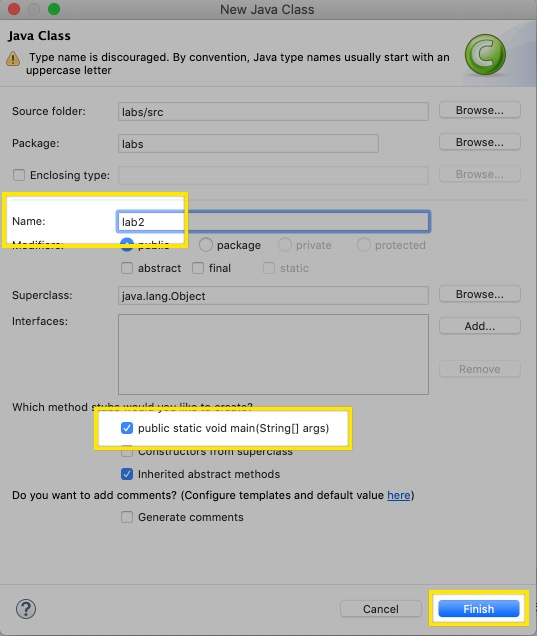## Writing Static Methods

Copy the folowing code inside the `public class lab2 {` in the project we just created:

``````public static void print3Times(String s) {
for (int i = 0; i < 3; i++) {
System.out.println(s);
}
}``````

The static method called print3Times that takes a string as its parameter and prints it three times.
Note: The header of the method has the word void in it. Make sure you understand what that means, and ask us if you’re not sure.

Note how this is a static method. Becuase of this, to call the code in the main method all we need to do is add `print3Times("test");`to the main function and we get the desired result: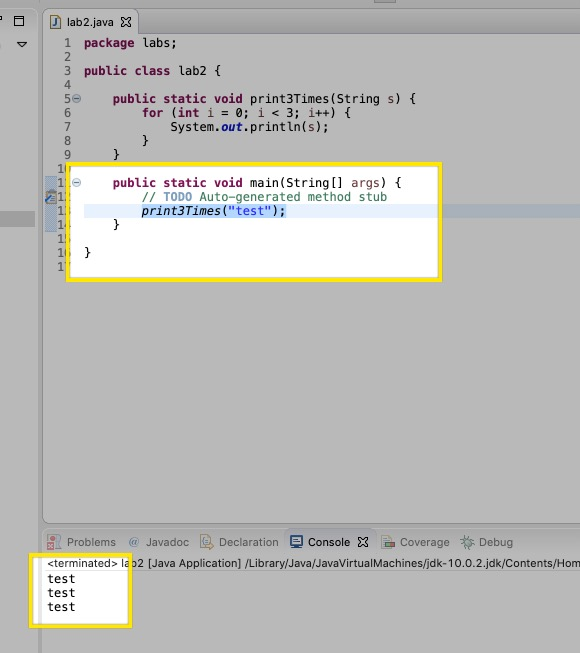In this lab we'll see how this is very different from object methods.

## Working with Roman Numerals

Recall that a long time ago, numbers were represented via Roman Numerals. The values of each symbols are as follows

• I = 1
• V = 5
• X = 10
• L = 50
• C = 100
• D = 500
• M = 1000

Here are some rules to follow when converting from Roman Numerals to Digits

1. Reading left to right symbols with higher values will generally appear first. The overall value is given by taking the sum of values from left to right
2. If a single lower value is placed left of a higher value letter, it is subtracted from the total instead.
3. Only one single lower value can be subtracted from a higher value
4. ”I” can only be subtract from ”V” and ”X”. ”X” can only subtract from ”L” and ”C”, ”C” can only subtract from ”D” and ”M’, and ”V”, ”L”, and ”D” can never subtract.

## Part 1: Static Roman Numerals

Copy the folowing code inside the `public class lab2 {` in the project we just created:

`````` private static int convertSingleRomanNumeral(char numeral) {
int val = 0;

if(numeral == 'I') {
val = 1;
} else if(numeral == 'V') {
val = 5;
} else if(numeral == 'X') {
val = 10;
} else if(numeral == 'L') {
val = 50;
} else if(numeral == 'C') {
val = 100;
} else if(numeral == 'D') {
val = 500;
} else if(numeral == 'M') {
val = 1000;
}

return  val;
}

public static int convert(String romanNum) {

int numericalValue = 0;

for(int i = 0 ; i < romanNum.length() - 1 ; i++) {
if ( convertSingleRomanNumeral(romanNum.charAt(i)) <
convertSingleRomanNumeral(romanNum.charAt(i+1)) ) {
numericalValue -= convertSingleRomanNumeral(romanNum.charAt(i));
} else {
numericalValue += convertSingleRomanNumeral(romanNum.charAt(i));
}
}
return numericalValue + convertSingleRomanNumeral(romanNum.charAt(romanNum.length()-1));
}

public static int add(String romanNum1, String romanNum2) {
return convert(romanNum1) + convert(romanNum2);
}``````

First: Lets test out the convert method by calling it in the main function. Try to figure it out yourself and we'll go over it as a class. Try printing the following Roman Numberals:
Note: Think about what the convert function returns and how we print things to the console.

• X
• LXXXXIX
• CDV

We should get an output like this: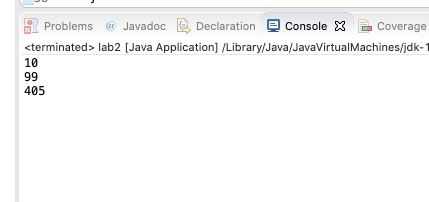Second: Lets try out add method by calling it in the main method. Try to figure it out yourself and we'll go over it as a class. Try adding the following Roman Numberals:

• X + X
• XI + CDV
• LXXXXIX + I

We should get an output like this: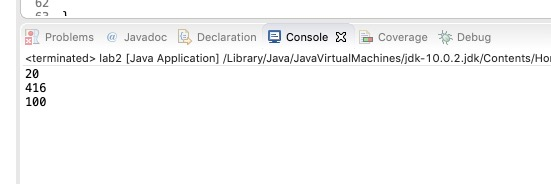## Part 2: Roman Numeral Objects

Classes made up of only static methods are good for something, but don't take full advantage of Object Oriented Paradigm (OOP) that java is based on. To illustrate the difference we will convert our static Roman Numeral API into an actual object class.

1. First lets create a new class called RomanNumeral
2.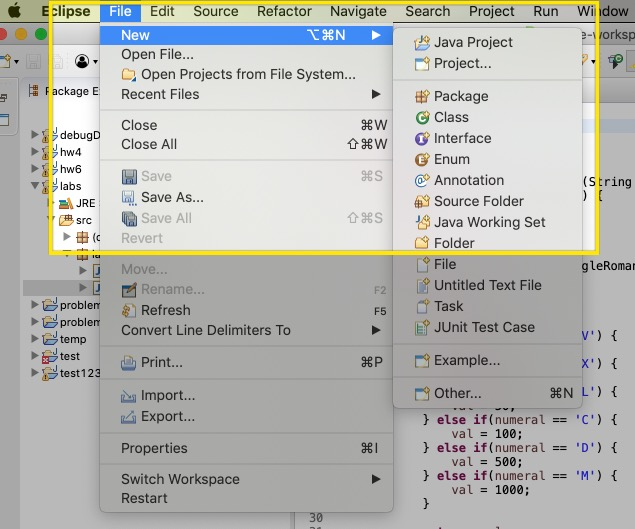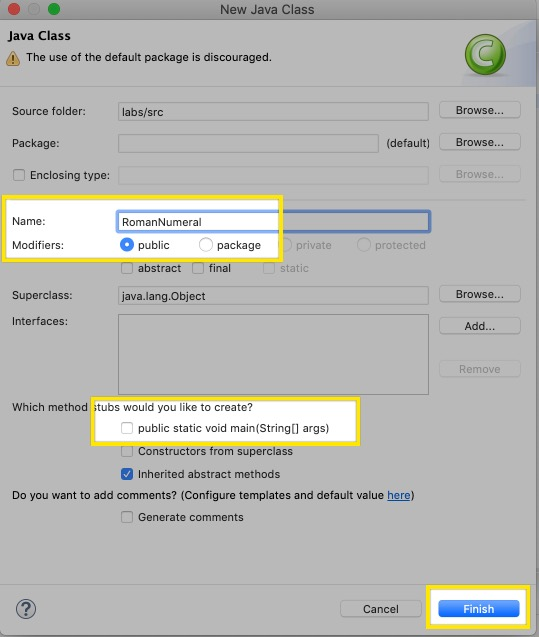3. Lets think about what our object will have to store. We're going to have to keep the numerical value and the string value of our Roman numeral. Lets add that to our object
4.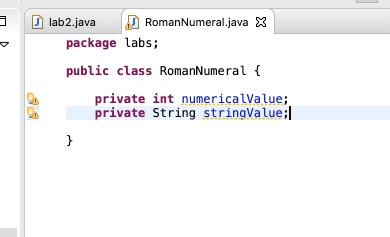5. Now lets add a constructor. This will be used to first initialize our object
6.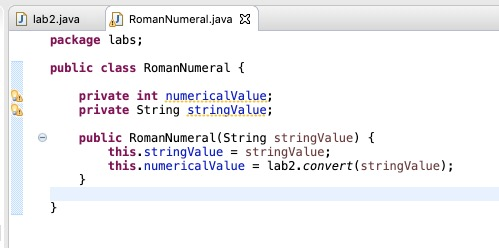We can see now when we first create our object it will take in a string (i.e. "XI") and convert that strinig and store it in the numerical value field of out object.

Now to finish the lab create the following methods, the headers are given:

• Create the to string method that gives back the Roman Numeral representation of this number (i.e. String value)
• `public String toString()`
• Create an equals method that checks if 2 RomanNumerals are equivalent
• `public boolean equals(RomanNumeral other)`
• Create an add method that takes in another RomanNumeral object, and returns an integer that is the sum of the invoked RomanNumeral, and the other RomanNumeral
• `public int add(RomanNumeral other)`

``````RomanNumeral x = new RomanNumeral("X");